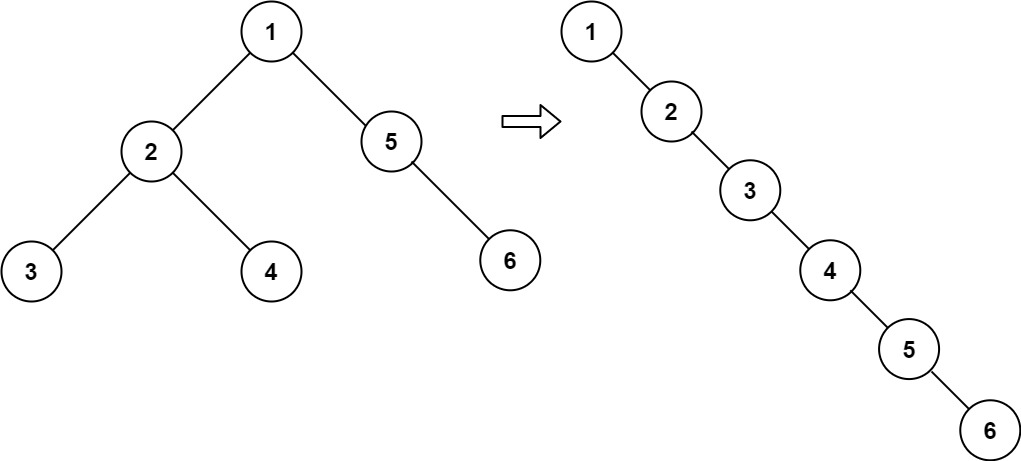# GeetCode Hub

Given the `root` of a binary tree, flatten the tree into a "linked list":

• The "linked list" should use the same `TreeNode` class where the `right` child pointer points to the next node in the list and the `left` child pointer is always `null`.
• The "linked list" should be in the same order as a pre-order traversal of the binary tree.

Example 1:```Input: root = [1,2,5,3,4,null,6]
Output: [1,null,2,null,3,null,4,null,5,null,6]
```

Example 2:

```Input: root = []
Output: []
```

Example 3:

```Input: root = 
Output: 
```

Constraints:

• The number of nodes in the tree is in the range `[0, 2000]`.
• `-100 <= Node.val <= 100`

Follow up: Can you flatten the tree in-place (with `O(1)` extra space)?

/** * Definition for a binary tree node. * public class TreeNode { * int val; * TreeNode left; * TreeNode right; * TreeNode() {} * TreeNode(int val) { this.val = val; } * TreeNode(int val, TreeNode left, TreeNode right) { * this.val = val; * this.left = left; * this.right = right; * } * } */ class Solution { public void flatten(TreeNode root) { } }